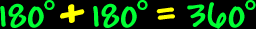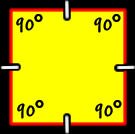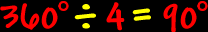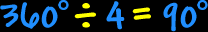Polygons:  Properties of QuadrilateralsSum of the Interior Angles of Quadrilaterals:To find the sum of the interior angles of a quadrilaterals, divide it up into triangles... There are two triangles...  Because the sum of the angles of each triangle is 180 degrees...  We getSo, the sum of the interior angles of a quadrilateral is 360 degrees.Regular Quadrilaterals - Squares: The properties of squares:All sides are the same length (congruent) and all interior angles are the same size (congruent).To find the measure of the interior angles, we know that the sum of all the angles is 360 degrees (from above)...  And there are four angles...So, the measure of the interior angle of a square is 90 degrees.The measure of the central angles of a square:To find the measure of the central angle of a square, make a circle in the middle...  A circle is 360 degrees around...  Divide that by four angles...So, the measure of the central angle of a square is 90 degrees.